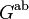# Exact sequence giving kernel of mapping from tensor square to exterior square

## Statement

Let$G$ be any group. There is a natural exact sequence as follows:$H_3(G;\mathbb{Z}) \to \Gamma(G^{\operatorname{ab}}) \to G \otimes G \to G \wedge G \to 0$

Here:

•$H_3(G;\mathbb{Z})$ denotes the third homology group for trivial group action of$G$ on$\mathbb{Z}$.
•$\Gamma$ denotes the universal quadratic functor and$G^{\operatorname{ab}}$ denotes the abelianization of$G$.
•$G \otimes G$ denotes the tensor square of$G$, i.e., the tensor product of$G$ with itself where both parts of the compatible pair of actions are the action of$G$ on itself by conjugation.
•$G \wedge G$ is the exterior square of$G$.

The maps are as follows:

• The first map is mysterious (some kind of connecting homomorphism?)
• The second map,$\Gamma(G^{\operatorname{ab}}) \to G \otimes G$, sends PLACEHOLDER FOR INFORMATION TO BE FILLED IN: [SHOW MORE]
• The third map,$G \otimes G \to G \wedge G$, is the natural quotient map that sends a generator of the form$x \otimes y$ to the corresponding element$x \wedge y$.